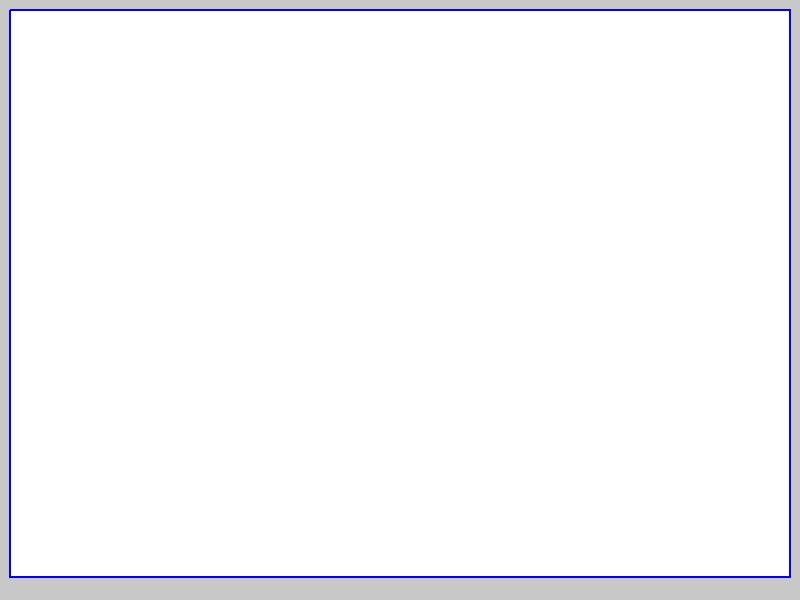### Approximation by polynomial function.

by Leonid Sakharov

A demonstration of PHP codes for approximation of the curve by polynomial function.Fitting formula: A fit of data series with records with polynomial formula of 2 grade.
= 0 +0*

Coefficient of correlation: 1.0000
Fitting coefficients:
 Grade Coefficient Standard deviation Correlation coefficient, % 0 0 0 0.00 1 0 0 0.00

Paste or type data:
Click on button to get sample data:

Degree of polynom:

Jun. 1, 2017; 09:46 EST